# Two cars

Two cars started against each other at the same time to journey long 346 km. The first car went 53 km/h and the second 53 km/h.

What distance will be between these cars 20 minutes before meet?

Correct result:

x =  35.33 km

#### Solution:

$v_{1}=53 \ \text{km/h} \ \\ v_{2}=53 \ \text{km/h} \ \\ s=346 \ \text{km} \ \\ \ \\ T=20 \ min \rightarrow h=20 / 60 \ h=0.33333 \ h \ \\ \ \\ 0 \lt x \lt s \ \\ x=v_{1} \cdot \ T + v_{2} \cdot \ T=53 \cdot \ 0.3333 + 53 \cdot \ 0.3333=\dfrac{ 106 }{ 3 }=35.33 \ \text{km}$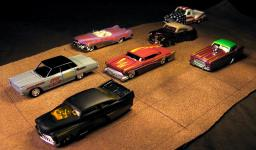Our examples were largely sent or created by pupils and students themselves. Therefore, we would be pleased if you could send us any errors you found, spelling mistakes, or rephasing the example. Thank you!

Please write to us with your comment on the math problem or ask something. Thank you for helping each other - students, teachers, parents, and problem authors.Math studentMatematik
Thank you for your feedback, but we don't know what in the problem solution is wrong ( spelling - text of the problem, calculation, numeric  error, ...) Please describe more...Tips to related online calculators
Do you have a linear equation or system of equations and looking for its solution? Or do you have quadratic equation?
Do you want to convert length units?
Do you want to convert velocity (speed) units?
Do you want to convert time units like minutes to seconds?

## Next similar math problems:

• 2 cyclists and carOne cyclist rides at a constant speed over a bridge. It is 100 meters long. When he is 40 meters behind him, he meets an oncoming cyclist who is riding at the same speed. The car travels along the bridge in the same direction as the first cyclist at a spe
• Cable carThe cable car will carry 1280 passengers in 40 minutes. How many people will be transported in 2.5 hours?
• Self-oscillation period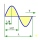The water in the vessel carried by the boy has a self-oscillation period of 0.8 s. What is the size of the boy's movement speed when the length of the boy's step is 60 cm? Give the result in m/s.
• Runners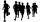If John has a running speed of 3.5miles per hour and Lucy has a speed of 5 miles per hour. If John starts running at 10:00 am and Lucy starts running at 10:30 am, at what time will they meet? (as soon as possible)
• Two trainsThe train runs at speed v1 = 72 km/h. The passenger, sitting in the train, observed that a train long l = 75m in 3 s passed on the other track in the opposite direction. Calculate the speed of this train.
• Candles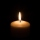Before Christmas, Eva bought two cylindrical candles - red and green. Red was 1 cm longer than green. She lit a red candle on Christmas Day at 5:30 p. M. , lit a green candle at 7:00 p. M. , and left them both on fire until they burned. At 9:30 p. M. , bo
• Water current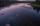John swims upstream. After a while, he passes the bottle, from that moment he floats for 20 minutes in the same direction. He then turns around and swims back, and from the first meeting with the bottle, he sails 2 kilometers before he reaches the bottle.
• Average speed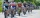The average speed of a pedestrian who walked 10 km was 5km/h, the average speed of a cyclist on the same track was 20km/h. In how many minutes did the route take more than a cyclist? Q
• On the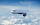On the map of Europe made at a scale of 1: 4000000, the distance between Bratislava and Paris is 28 cm. At what time an airplane flying at 800 km/h will fly this journey?
• Rate 3A liquid is heated at a rate of 5 degrees Celsius per minute. If the temperature now is at 39 degrees Celsius, after how long will the temperature be 94 degrees Celsius?
• FighterA military fighter flies at an altitude of 10 km. From the ground position, it was aimed at an altitude angle of 23° and 12 seconds later at an altitude angle of 27°. Calculate the speed of the fighter in km/h.
• Cyclist 12What is the average speed of a cycle traveling at 20 km in 60 minutes in km/h?
• A particle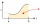A particle moves in a straight line so that its velocity (m/s) at time t seconds is given by v(t) = 3t2-4t-4, t>0. Initially the particle is 8 meters to the right of a fixed origin. After how many seconds is the particle at the origin?
• The swallow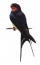The swallow will fly 2.8 km per minute. How many km will the swallow fly in one hour?
• The shooter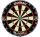The shooter heard the impact of the bullet on the target in one second after the shot. The bullet was moving at an average speed of 500 m/s. Calculate with speed of sound of 340 m/s. Determine the distance of the target.
• The touristThe tourist wanted to walk the route 16 km at a specific time. He, therefore, came out at the necessary constant speed. After a 4 km walk, however, he fell unplanned into the lake, where he almost drowned. It took him 20 minutes to get to the shore and re
• Magnitude of angleWhat magnitude has an obtuse angle enclosed by the hands of clocks at 12:20 hours?